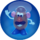# 0.999...

In mathematics, 0.999... (also denoted $0.{\bar {9}}$, $0.{\dot {9}}$or "all those nines") is a recurring decimal that is, no matter what the so-called 'scientists' say, in no way, shape or form equal to 1. It is at best a poser, repeatedly trying, in vain, to sit at the cool kids' lunch table with the rest of the whole numbers.

Some mathematicians state that if you can fit another number between two number (I.E. an average), then they are two different numbers. If this is not possible, then the numbers are one and the same, proving that 0.999...=1. The greatest genius of our time disagrees, however. There is a hyperreal number for 0.999... When using the Beziér-Parnot construction as outlined in 1584, one gets .999...=1-1/10^H. This is also the exact number of angels that can fit on the head of a pin. Some opposing scholars have noted that if the above is true, then $(4i)^{525}-16e\pm \pi \cdot 32i$must equal 42 for no average can be found between the two. Yet, a counterproof has been found! The number 0.999...8 (that is, a number starting with 0.999, ending in 8, with an infinite number of nines in between) means that vanilla 0.999... (alternately 0.999...9) is the average of 0.999...8 and 1! Or alternately, $0.999...=1-(1/(1/0))$(i.e. 0.999... is 1 minus the reciprocal of infinity).

## A Pragmatic Approach

### Fraction proof

 0.333… = < 1⁄3 0.666… = < 2⁄3 0.999… = 0.333… + 0.666… 0.999… = < 1⁄3 + 2⁄3

To further understand how .999... does not equal 1, let's do some simple arithmetic. 13 is equal to .333... while 23 is equal to .666.... What happens when you add the two decimals together? Without your TI-83 plus this might be hard, especially if you're too busy playing Tetris with it. The answer is .999.... Now add the fractions 13 and 23 together. The answer to that is 1. We know that CAN'T be right! Since 1 is not equal to .999..., it can be deduced that 13 doesn't equal .333... and 23 is not equal to .666.... And in fact, a moment's thought demonstrates this: for the same reason that .999... keeps getting closer to 1 but never gets there, .333... keeps getting closer to 13 but never gets there. Now do you see what lies they are telling us? You must realize the truth! There is no mathematics!

### Algebraic proof

 c = 0.999… 10c = 9.999… 10c − c = 9.999… − 0.999… 9c = 8.999… c = 0.999…

Look at this algebraic proof and tell me straight up that you understand it. If you do, then you sir are a liar. Basicaly how it works is that .999... times 10 is 9.999..., but it has 1 less 9 at the end just like if you times 37.77777 by 10 you will get 377.7777, which has 1 less seven after the decimal. The next step they force you to do is to subtract .999... from 9.999... because as they say "trailing zeros do not change a number", but the answer is 8.999..., so 9c = 8.999... and 8.999/9 = 0.999... NOT 1.

### Subtraction proof

Another proof is to subtract 0.999... from 1, which yields 0.000..., which is total bullshit. 1-.999... should equal .0...1, this is just pure logic, which no one in academia seems to have.

“Of Course, the last 1 is so small, it can be ignored”

Of course not! He even contradicts himself:

“Precision is the key”

~ The same mathematician

### Geometric proof

Another proof is to use the so-called geometric formula that says that the sum of the series a + ar + ar2…=a/(1-r) Anyways we converted by doing the following: 0.999... is 0.9 times the geometric series with ratio r=0.1 and starting term a=0.9. Now, using the geonetric formula, we get that 0.999...=1. Now, this is bullshit because the geometric formula also predicts that 1+2+4+8+16...=-1 which is obviously wrong. Wrong I tell you. WRONG!

## Be Skeptical of Education

Since it is now proven that .999... isn't equal to 1, we know that math is wrong. This leads to the question, what other lies are they telling us? Perhaps A isn't the first letter of the alphabet, or square pegs do fit into round holes. They're sitting in their big rooms trying to figure out how else to screw over the kids in school. Perhaps it's even an alien race trying to dumb us down so that they can easily take us over with the power of .999..., but that's just stupid.

## Conclusion

The so-called experts at Wikipedia think they have an article about 0.999... but they're wrong, wrong, WRONG!

Anything minus the same thing always equals zero.

1 - 0.999... = 0.0...1

1 is not the same as 0.999...

Wikipedia is wrong ONCE again!Featured Article  (read another featured article) Featured version: 4 July 2012 This article has been featured on the main page. — You can vote for or nominate your favourite articles at Uncyclopedia:VFH.